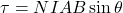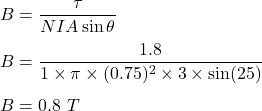Question

A single circular loop of wire of radius 0.75 m carries a constant current of 3.0 A. The loop may be rotated about an axis that passes through the center and lies in the plane of the loop. When the orientation of the normal to the loop with respect to the direction of the magnetic field is 25°, the torque on the coil is 1.8 N ⋅ m. What is the magnitude of the uniform magnetic field exerting this torque on the loop?

1.ngocdiep

B = 0.8 T

Explanation:

It is given that,

Radius of circular loop, r = 0.75 m

Current in the loop, I = 3 A

The loop may be rotated about an axis that passes through the center and lies in the plane of the loop.

When the orientation of the normal to the loop with respect to the direction of the magnetic field is 25°, the torque on the coil is 1.8 Nm.

We need to find the magnitude of the uniform magnetic field exerting this torque on the loop. Torque acting on the loop is given by :B is magnetic fieldSo, the magnitude of the uniform magnetic field exerting this torque on the loop is 0.8 T.